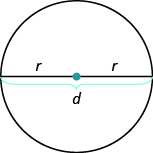## Key Concepts

• Problem Solving Strategy for Geometry Applications
1. Read the problem and make sure you understand all the words and ideas. Draw the figure and label it with the given information.
2. Identify what you are looking for.
3. Name what you are looking for. Choose a variable to represent that quantity.
4. Translate into an equation by writing the appropriate formula or model for the situation. Substitute in the given information.
5. Solve the equation using good algebra techniques.
6. Check the answer in the problem and make sure it makes sense.
7. Answer the question with a complete sentence.
• Properties of Circles• $d=2r$
• Circumference: $C=2\pi r$ or $C=\pi d$
• Area: $A=\pi {r}^{2}$

## Glossary

irregular figure
An irregular figure is a figure that is not a standard geometric shape. Its area cannot be calculated using any of the standard area formulas.

## Contribute!

Did you have an idea for improving this content? We’d love your input.